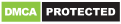### 1. Consider the following statements associated with the laws of weights in the theory of erros : 1. If an equation is multiplied by its own weight, then the weight of the resulting equation is equal to the reciprocal of the weight of the equation. 2. The weight of the algebraic sum of two or more quantities is equal to the reciprocal of the sum of the individual weights. 3. If the quantity of a given weight is multiplied by a factor, then the weight of the result is obtained by dividing its given weight by the square root of that factor. 4. If the quantity of a given weight is divided by a factor, then the weight of the result is obtained by multiplying its given weight by the square of that factor. Of these statements :

Terms And Service:We do not guarantee the accuracy of available data ..We Provide Information On Public Data.. Please consult an expert before using this data for commercial or personal usePowered By:Omega Web Solutions
© 2002-2017 Omega Education PVT LTD...Privacy | Terms And Conditions